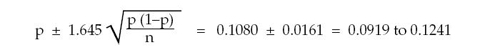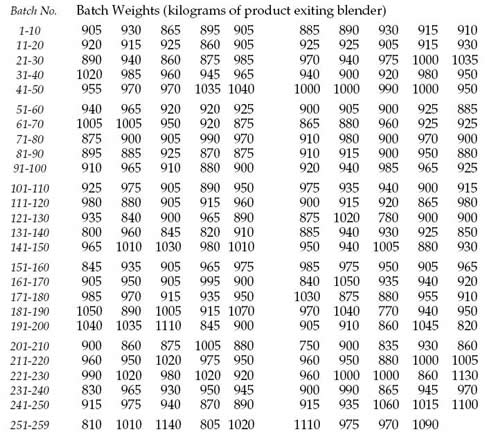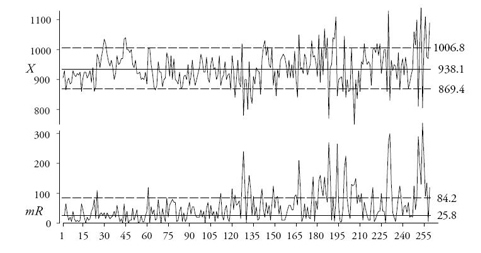#Quality Digest

Featured Product
This Week in Quality Digest Live
Six Sigma Features
Donald J. Wheeler
In spite of what everyone says to the contrary
Brittney McIver
Every CAPA should begin with investigation
Anthony Tarantino
Smart technologies provide a single source of truth for rapid response and accurate decision-making
Scott A. Hindle
To understand the signals in your data you need to know how they were collected
V R Vijay Anand
Reduce waste by improving efficiency, enabling predictive maintenance, and streamlining resource management
Six Sigma News
Elsmar Cove is a leading forum for quality and standards compliance
Is the future of quality management actually business management?
Too often process enhancements occur in silos where there is little positive impact on the big picture
Collect measurements, visual defect information, simple Go/No-Go situations from any online device
Good quality is adding an average of 11 percent to organizations’ revenue growth
Floor symbols and decals create a SMART floor environment, adding visual organization to any environment
A guide for practitioners and managers
Making lean Six Sigma easier and adaptable to current workplaces
Gain visibility into real-time quality data to improve manufacturing process efficiency, quality, and profitsBio

Six Sigma

## The Imaginary Theorem of Large Samples

### How many data samples do you need?

Published: Monday, April 5, 2010 - 10:10

Courses in statistics generally emphasize the problem of inference. In my December column, “The Four Questions of Data Analysis,” I defined this problem in the following manner:

Given a single unknown universe, and a sample drawn from that universe, how can we describe the properties of that universe?

In general, we attempt to answer this question by estimating characteristics of the universe using statistics computed from our sample.One of the lessons that most students of statistics manage to learn is that, in questions of inference, the uncertainty of an estimator is inversely related to the amount of data used. To illustrate this relationship, I will draw beads from my bead box. A paddle with holes on one side is used to obtain a sample of 50 beads. The number of yellow beads in each sample of 50 beads is recorded. The beads are replaced in the bead box, the beads are stirred up, and the whole sequence begins again. After 10 such drawings, I have drawn 500 beads and have found a total of 65 yellow beads.

My point estimate for the proportion of yellow beads in the bead box is thus:

p = 65/500 = 0.1300 (or 13%)

and the usual 90-percent interval estimate for the proportion of yellow beads is:I repeat the experiment and find 43 yellow beads out of 500 beads sampled. Combining the results from both experiments we have a point estimate for the proportion of yellow beads in the bead box of:

p = 108/1000 = 0.1080 (or 10.8%)

and the usual 90-percent interval estimate for the proportion of yellow beads is:While the point estimate changed, the element of interest here is how the uncertainty decreased from 0.0247 to 0.0161 as we went from using 10 samples to using 20 samples in our estimate. With increasing amounts of data our estimates come to have lower levels of uncertainty. Figure 1 shows the results of 20 repetitions of this experiment of drawing 10 samples of 50 beads each from my bead box. The second column gives the cumulative number of yellow beads. The third column gives the cumulative number of beads sampled. The fourth column lists the cumulative point estimates of the proportion of yellow beads, while the last two columns list the end points for the 90-percent interval estimates for the proportion of yellow beads. Figure 2 shows these last three columns plotted against the number of the experiment which is listed in the first column of figure 1.Figure 1: Twenty bead box experimentFigure 2: Cumulative proportion yellow and 90% interval estimates

As we look at figure 2, we see the point estimate converge on a value near 0.11 and stabilize there while the uncertainty keeps dropping and the interval estimate gets tighter and tighter. This is the picture shown in textbook after textbook, and the source of the theorem of large samples. Based on this graph, it would appear that this experiment will yield an average of 11-percent yellow beads.

But is this a reasonable estimate of the proportion of yellow beads in my bead box? Because there are only 4,800 beads in the box, the 20 repetitions of our experiment effectively looked at every bead in the box twice. Yet, by actual count, the box contains only 10-percent yellow beads, a value that was outside the interval estimate from experiment No. 5 on. As we collected more and more data our point estimate did converge, but it did not converge to the “true value.”

So here we come to the first problem with the theorem of large samples. The whole body of computations involved with estimation are built on certain assumptions. One of these is the assumption that we have drawn random samples from the universe. However, random samples are nothing more than a concept. There is no rigorous mathematical definition of random. In practice, we always have to use some sort of sampling system or sampling device. Here we used mechanical sampling. And regardless of how careful we may be, mechanical sampling is not the same as the assumption of random sampling.

Here we ended up with an excess number of yellow beads in our samples. Nothing in our computations can compensate for this bias. Moreover, in practice, where we cannot stop the experiment and find the “true value” by counting all the beads, there will be no way to even detect this bias. Thus, in practice, the first problem with the theorem of large samples is that, because of the way we obtain our data, our estimates may not converge to the values that we expect.

If this problem is not enough to give you pause, there is an even bigger problem with the theorem of large samples.

To illustrate this second problem, I shall use the batch weight data shown in figure 3. There you will find the weights, in kilograms, of 259 successive batches produced during one week at a plant in Scotland. For purposes of this example, assume that the specifications are 850 kg to 990 kg. After every tenth batch, the capability ratio is computed using all of the data for that week. Thus, just like the proportion of yellow beads, we would expect to see these capability ratios converge to some value as the uncertainty drops with the increasing amounts of data used. Figure 4 shows the number of batches used for each computation, the capability ratios found, and the 90-percent interval estimates for the process capability. These values are plotted in sequence in figure 5.Figure 3: The batch weight dataFigure 4: Cumulative estimates of capability ratio for batch weight dataFigure 5: Cumulative estimates of capability ratio for the batch weight data

There we see that these capability ratios do not converge to any one value. Instead they meander over time. While the uncertainties decrease with increasing amounts of data, this reduction in uncertainty is meaningless when the target itself is uncertain. We get better and better estimates of something, but that something may have already changed by the time we have the estimate.

To understand the behavior of these capability ratios we need to look at the XmR chart for these data in figure 6. The limits shown are based on the first 60 values. This baseline was chosen to obtain a reasonable characterization of the process potential. Here we see a process that is not only unpredictable, but one that gets worse as the week wears on.Figure 6: XmR chart for the batch weight data

The theorem of large samples implicitly assumes that there is one universe. When there are multiple universes, and these universes are changing around without warning, no amount of data will ever be sufficient to provide a good estimate of any process characteristic.

This takes us back to the question of homogeneity, which is the fundamental question of data analysis. Did these data come from a process or system that appears to be operating in the same way over time? Or do these data show evidence that the underlying process has changed in some manner while the data were collected? The only statistical technique that can answer this question of homogeneity is the process behavior chart.

If the process behavoir chart doesn’t show any evidence of a lack of homogeneity, then our process may be predictable, and the theorem of large samples may only suffer from the problem of estimating the wrong thing. (Drawings out of a bead box are a classic example of a predictable process.)

But if we find evidence of a lack of homogeneity within our data, then we know that our process has a multiple personality disorder, and any attempt to use the theorem of large samples is merely wishful thinking—no amount of data will ever be sufficient to provide a reliable estimate of a process parameter.

### Discuss### Donald J. Wheeler

Find out about Dr. Wheeler’s virtual seminars for 2022 at www.spcpress.com. Dr. Wheeler is a fellow of both the American Statistical Association and the American Society for Quality who has taught more than 1,000 seminars in 17 countries on six continents. He welcomes your questions; you can contact him at djwheeler@spcpress.com.

I have always read with the gratest interest your articles. In this case, I have doubts that 2 samples of size 500 each is the same as 1 sample of size 1000. In fact, if you calculate the proportion for each of the samples and the interval estimate for the population proportion, we get:
Experiment 1 2 3 4 5 6 7 8 9 10 11 12 13 14 15 16 17 18 19 20
# yellow cum 65 108 164 219 276 336 389 448 506 553 609 655 714 770 817 874 927 981 1040 1105
# yellow 65 43 56 55 57 60 53 59 58 47 56 46 59 56 47 57 53 54 59 65
Point estimate 0.130 0.086 0.112 0.110 0.114 0.120 0.106 0.118 0.116 0.094 0.112 0.092 0.118 0.112 0.094 0.114 0.106 0.108 0.118 0.130
Low 0.105 0.065 0.089 0.087 0.091 0.096 0.083 0.094 0.092 0.073 0.089 0.071 0.094 0.089 0.073 0.091 0.083 0.085 0.094 0.105
High 0.155 0.107 0.135 0.133 0.137 0.144 0.129 0.142 0.140 0.115 0.135 0.113 0.142 0.135 0.115 0.137 0.129 0.131 0.142 0.155
0.1 inside? Fail Correct Correct Correct Correct Correct Correct Correct Correct Correct Correct Correct Correct Correct Correct Correct Correct Correct Correct Fail
where 2 out of the 20 intervals don't include the correct population proportion 0.1 wich completelly matches our 90% confidence interval.